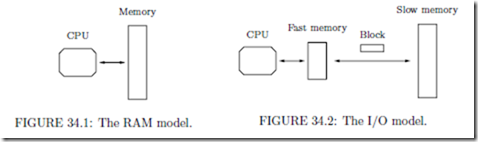# Cache-Oblivious Data Structures:The Cache-Oblivious Model

## The Cache-Oblivious Model

The memory system of most modern computers consists of a hierarchy of memory levels, with each level acting as a cache for the next; for a typical desktop computer the hierarchy consists of registers, level 1 cache, level 2 cache, level 3 cache, main memory, and disk. One of the essential characteristics of the hierarchy is that the memory levels get larger and slower the further they get from the processor, with the access time increasing most dramatically between main memory and disk. Another characteristic is that data is moved between levels in large blocks. As a consequence of this, the memory access pattern of an algorithm has a major inﬂuence on its practical running time. Unfortunately, the RAM model (Figure 34.1) traditionally used to design and analyze algorithms is not capable of capturing this, since it assumes that all memory accesses take equal time.

Because of the shortcomings of the RAM model, a number of more realistic models have been proposed in recent years. The most successful of these models is the simple two-level I/O-model introduced by Aggarwal and Vitter  (Figure 34.2). In this model the memory hierarchy is assumed to consist of a fast memory of size M and a slower inﬁnite memory, and data is transfered between the levels in blocks of B consecutive elements. Computationcan only be performed on data in the fast memory, and it is assumed that algorithms have complete control over transfers of blocks between the two levels. We denote such a transfer a memory transfer. The complexity measure is the number of memory transfers needed to solve a problem. The strength of the I/O model is that it captures part of the memory hierarchy, while being suﬃciently simple to make design and analysis of algorithms feasible. In particular, it adequately models the situation where the memory transfers between two levels of the memory hierarchy dominate the running time, which is often the case when the size of the data exceeds the size of main memory. Agarwal and Vitter showed that comparison based sorting and searching require Θ(SortM,B (N )) = Θ( N logM/B N ) and Θ(logB N ) memory transfers in the I/O-model, respectively . Subsequently a large number of other results have been obtained in the model; see the surveys by Arge  and Vitter  for references. Also see Chapter 27.

More elaborate models of multi-level memory than the I/O-model have been proposed (see e.g.  for an overview) but these models have been less successful, mainly because of their complexity. A major shortcoming of the proposed models, including the I/O-model, have also been that they assume that the characteristics of the memory hierarchy (the level and block sizes) are known. Very recently however, the cache-oblivious model, which assumes no knowledge about the hierarchy, was introduced by Frigo et al. . In essence, a cache-oblivious algorithm is an algorithm formulated in the RAM model but analyzed in the I/O model, with the analysis required to hold for any B and M . Memory transfers are assumed to be performed by an oﬀ-line optimal replacement strategy. The beauty of the cache-oblivious model is that since the I/O-model analysis holds for any block and memory size, it holds for all levels of a multi-level memory hierarchy (see  for details). In other words, by optimizing an algorithm to one unknown level of the memory hierarchy, it is optimized on all levels simultaneously. Thus the cache-oblivious model is eﬀectively a way of modeling a complicated multi-level memory hierarchy using the simple two-level I/O-model.

Frigo et al.  described optimal Θ(SortM,B (N )) memory transfer cache-oblivious algorithms for matrix transposition, fast Fourier transform, and sorting; Prokop also described a static search tree obtaining the optimal O(logB N ) transfer search bound . Subsequently, Bender et al.  described a cache-oblivious dynamic search trees with the same search cost, and simpler and improved cache-oblivious dynamic search trees were then developed by several authors [10, 12, 18, 25]. Cache-oblivious algorithms have also been developed for e.g. problems in computational geometry [1, 10, 15], for scanning dynamic sets , for lay- out of static trees , for partial persistence , and for a number of fundamental graph problems  using cache-oblivious priority queues [5, 16]. Most of these results make the so-called tall cache assumption, that is, they assume that M > Ω(B2); we make the same assumption throughout this chapter.

Empirical investigations of the practical eﬃciency of cache-oblivious algorithms for sorting , searching [18, 23, 25] and matrix problems  have also been performed. The overall conclusion of these investigations is that cache-oblivious methods often outperform RAM algorithms, but not always as much as algorithms tuned to the speciﬁc memory hierarchy and problem size. On the other hand, cache-oblivious algorithms perform well on all levels of the memory hierarchy, and seem to be more robust to changing problem sizes than cache-aware algorithms.

In the rest of this chapter we describe some of the most fundamental and representative cache-oblivious data structure results. In Section 34.2 we discuss two fundamental primitives used to design cache-oblivious data structures. In Section 34.3 we describe two cache-oblivious dynamic search trees, and in Section 34.4 two priority queues. Finally, in Section 34.5 we discuss structures for 2-dimensional orthogonal range searching.